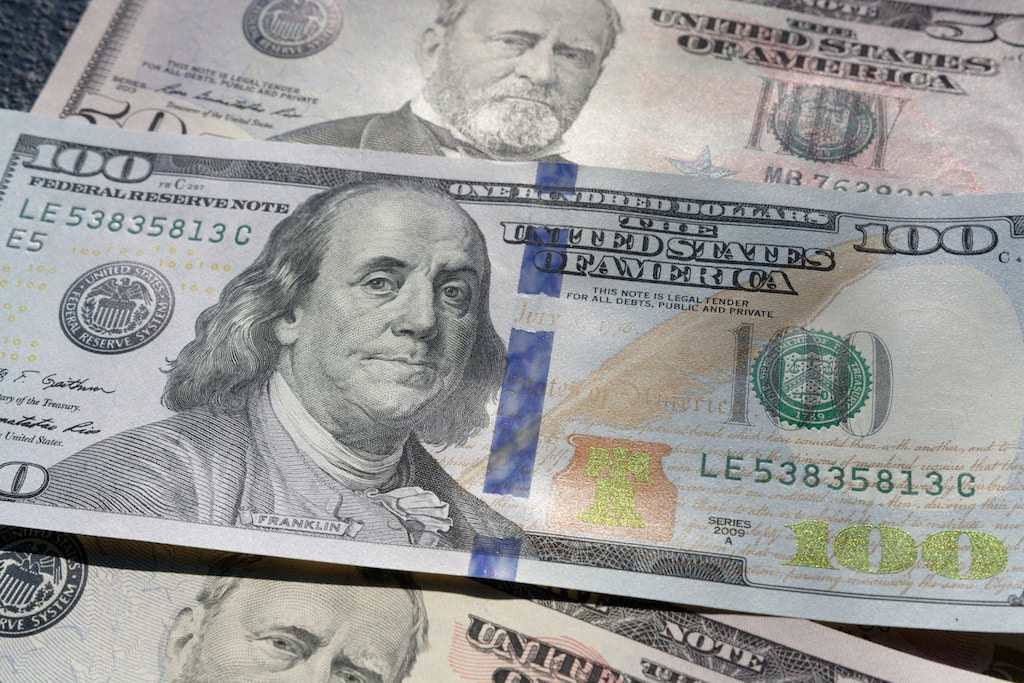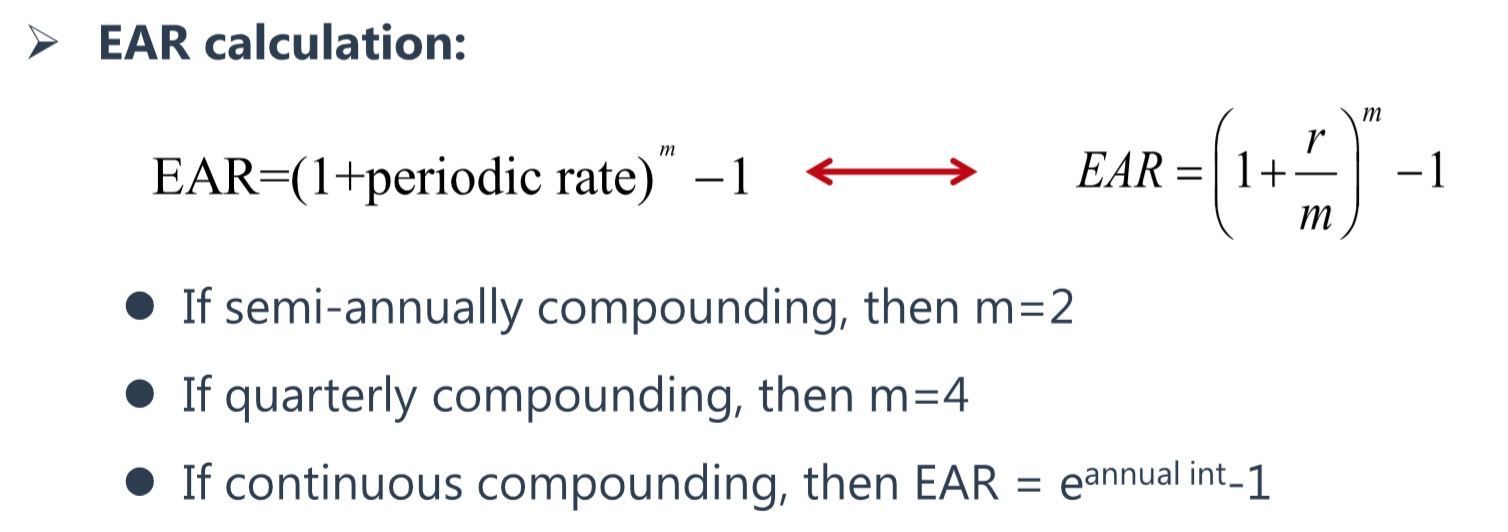(Photo by rawpixel on Unsplash)

##### 实际年利率换算公式

EAR=(1+R/t)^t-1

R：名义年利率

t：结息次数

EAR：实际年利率（Effective Annual Rate）

EAR=(1+3%/2)$^2$-1=3.0225%（二次方在网页上可能展示不出来）

##### CFA教材标准的EAR计算公式……

EAR=(1+3%/4)$^{4}$-1=3.033919%

……

EAR=(1+3%/12)$^{12}$-1=3.041596%

EAR=(1+3%/365)$^{365}$-1=3.045326%

……

##### 连续复利计算公式

EAR=$\lim_{t \to +\infty} (1 +\frac{R}{t})^t$-1=e$^R$-1

R：名义利率

t：结息次数

e：自然常数e)

EAR：实际年利率（Effective Annual Rate）

EAR=e$^{3\%}$-1=0.03045453=3.045453%

##### 参考资料